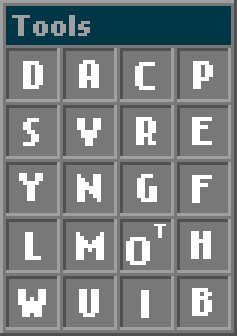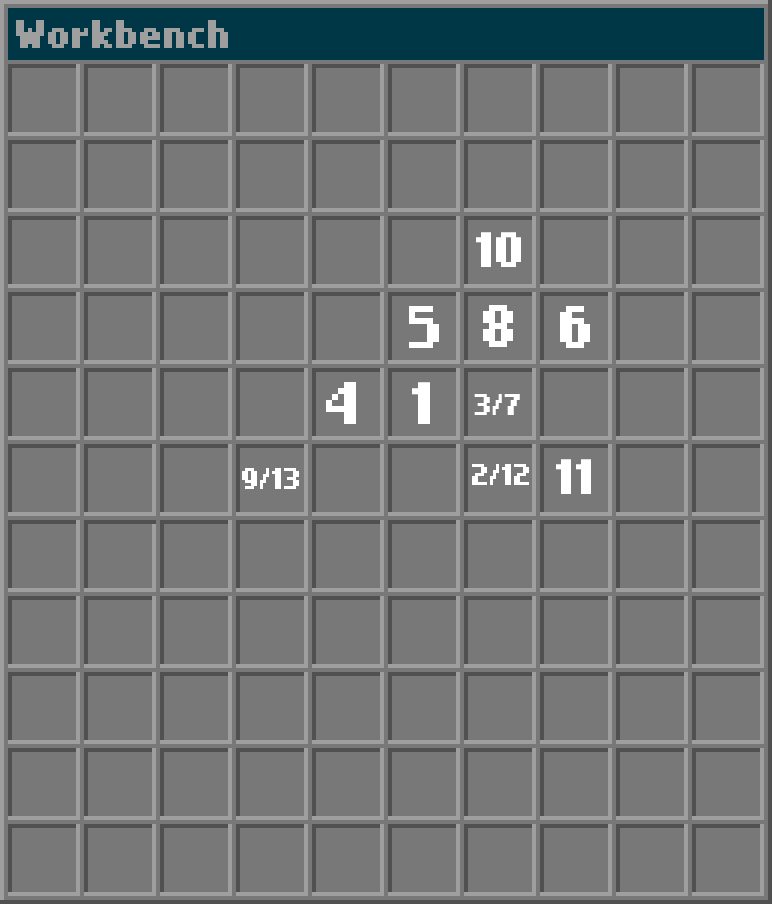# Holistic

The whole is greater than the sum of its parts.

1. How many solutions to this level are there?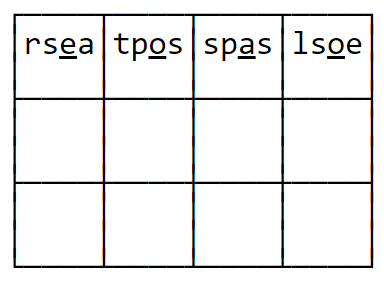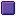) 1) 2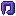) 3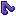) 4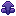) 5

2. Which of these animals cannot be placed on the grid?)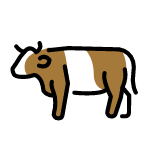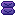)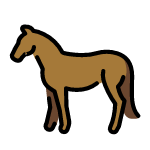)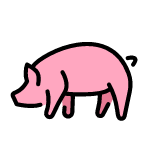)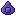)3. Which of these people does not appear in the city?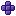)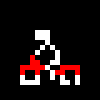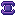)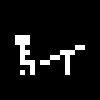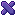)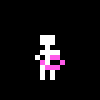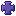))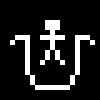4. You can add walls at exactly two of the labeled spaces to form a solvable level. Which two spaces?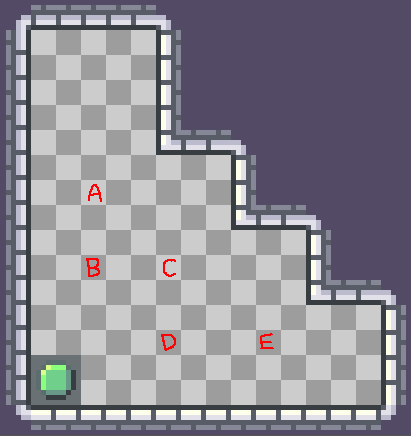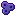) A and E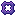) B and E) A and C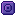) B and D) C and E

6. What is the minimum number of moves required to beat this game?) 49) 50) 52) 54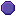) 56

7. What is the minimum number of players required to beat this game?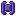) 4) 5) 6) 7) 8

8. Which of the following locations can be reached?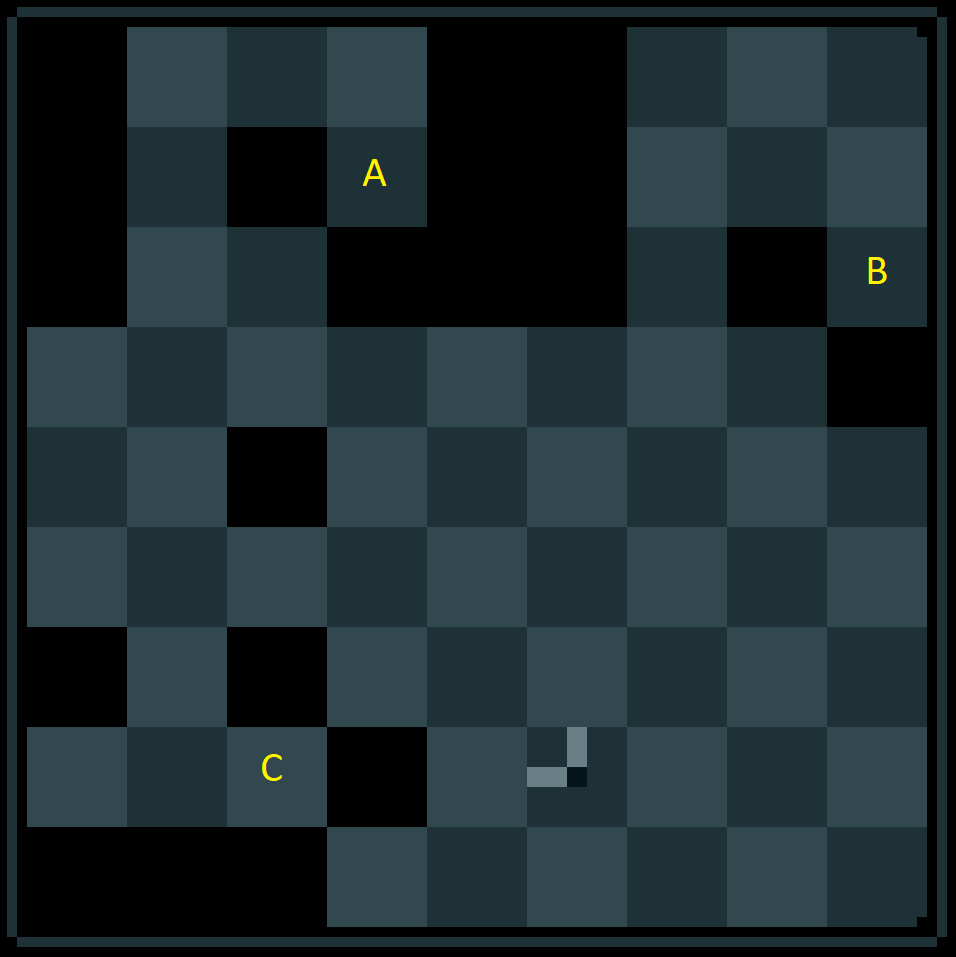) A only) C only) A and C) B and C) None of them

9. Which of the following levels are solvable? (You can press Enter to skip levels.)) 1 only) None of them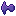) 1 and 2) 1 and 3) 1, 2, and 3

10. What is the minimum number of moves required to beat this level?) 25) 26) 27) 28) 30

11. Which of the following cups contain a ball?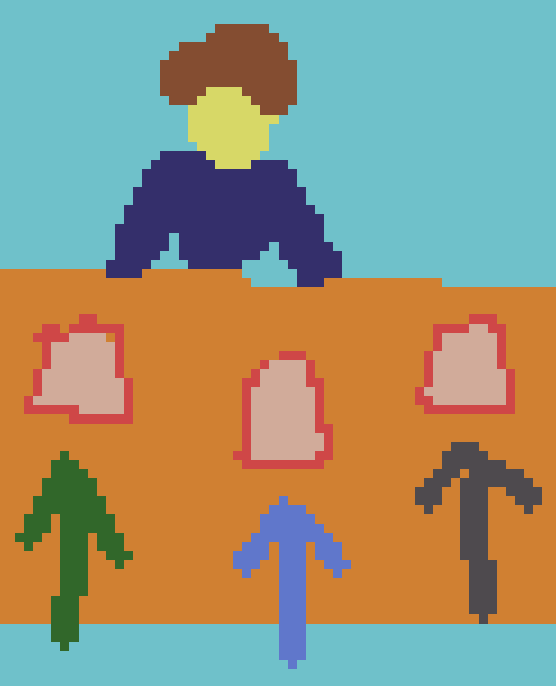) Left cup only) Middle cup only) Right cup only) Left and right cups) All three cups

12. What is the minimum number of moves required to beat this level?) 102) 103) 106) 110) 118

13. Which of the following levels are solvable?) 1, 2, and 3) 1 and 3) 1 and 2) 1 only) 3 only